Year 9 Interactive Maths - Second Edition

## Volume of a Triangular Prism

A triangular prism whose length is l units, and whose triangular cross-section has base b units and height h units, has a volume of V cubic units given by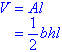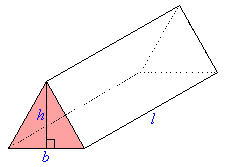#### Example 28

Find the volume of the triangular prism shown in the diagram.

##### Solution: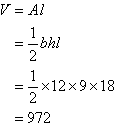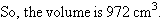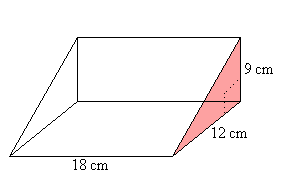triangular prism